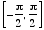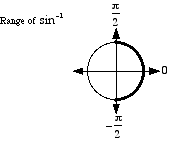# inverse sine

Inverse Sine
sin-1
Sin-1
arcsin
Arcsin

The inverse function of sine.

Basic idea: To find sin-1(½), we ask "what angle has sine equal to ½?" The answer is 30°. As a result we say sin-1(½) = 30°. In radians this is sin-1(½) = π/6.

More: There are actually many angles that have sine equal to ½. We are really asking "what is the simplest, most basic angle that has sine equal to ½?" As before, the answer is 30°. Thus sin-1(½) = 30° or sin-1(½) = π/6.

Details: What is sin-1(–½)? Do we choose 210°, –30°, 330° , or some other angle? The answer is –30°. With inverse sine, we select the angle on the right half of the unit circle having measure as close to zero as possible. Thus sin-1(–½) = –30° or sin–1(–½) = –π/6.

In other words, the range of sin-1 is restricted to [–90°, 90°] or.

Note: arcsin refers to "arc sine", or the radian measure of the arc on a circle corresponding to a given value of sine.

Technical note: Since none of the six trig functionssine, cosine, tangent, cosecant, secant, and cotangent are one-to-one, their inverses are not functions. Each trig function can have its domain restricted, however, in order to make its inverse a function. Some mathematicians write these restricted trig functions and their inverses with an initial capital letter (e.g. Sin or Sin-1). However, most mathematicians do not follow this practice. This website does not distinguish between capitalized and uncapitalized trig functions.See also

Copyrights © 2013 & All Rights Reserved by nafeducation.orghomeaboutcontactprivacy and policycookie policytermsRSS